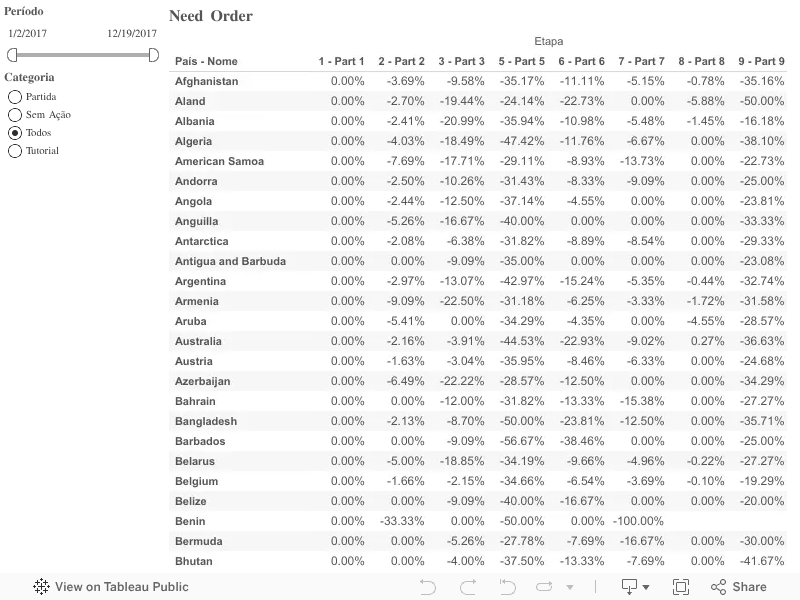2 Replies Latest reply on Dec 23, 2017 10:54 AM by Gabriel Fiorelli

# [How to Order] - Computed using table across measure

Hello.

First of all. Sorry for my not so good english. And also, it's the first time that i'm posting here.

Problem:

I'm trying to order by those columns values in that worksheet. But until now I wasn't able to do that.

Description:

The value in "2- Part 2" means that in this part there's -3,69% compared to the "1-Part 1".

The "3 - Part 3" have -9.58% compared to the "2 - Part 2". Following this pattern for each line.

But, the tableau function to order does not appear.

This field is computed by using Table (across).

The Measure is calculated by: (IF FIRST() = 0 THEN 0 ELSE (ZN(SUM([Players])) - LOOKUP(ZN(SUM([Players])), -1)) / ABS(LOOKUP(ZN(SUM([Players])), -1)) END)

The packaged workbook is attached.

Worksheet:• ###### 1. Re: [How to Order] - Computed using table across measure
2 of 2 people found this helpful
• ###### 2. Re: [How to Order] - Computed using table across measure

Thanks @Shinichiro Murakami, it was REALLY usefull.

It took some time to me understand, but it solve my problem, I was changing the entire data set to fit in a situation where I could sort the data. This solution saved my time.

Thanks again.
Fiorelli.